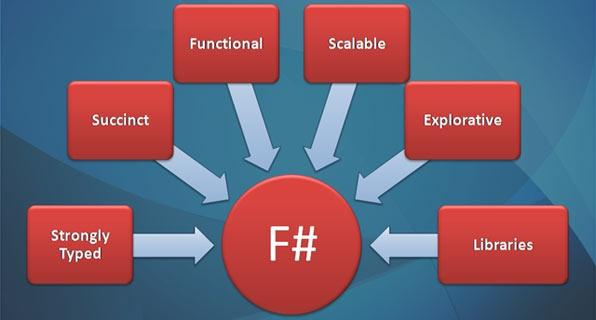JavaScript函数式编程（一）

一、容器、Functor

``````var foo = \$('#foo');
foo == document.getElementById('foo');
//=> false

foo == document.getElementById('foo');
//=> true
``````

``````var Container = function(x) {
this.__value = x;
}
Container.of = x => new Container(x);

//试试看
Container.of(1);
//=> Container(1)

Container.of('abcd');
//=> Container('abcd')
``````

``````Container.prototype.map = function(f){
return Container.of(f(this.__value))
}
``````

``````Container.of(3)
.map(x => x + 1)                //=> Container(4)
.map(x => 'Result is ' + x);    //=> Container('Result is 4')
``````

Functor（函子）是实现了 map 并遵守一些特定规则的容器类型。

``````var Maybe = function(x) {
this.__value = x;
}

Maybe.of = function(x) {
return new Maybe(x);
}

Maybe.prototype.map = function(f) {
return this.isNothing() ? Maybe.of(null) : Maybe.of(f(this.__value));
}

Maybe.prototype.isNothing = function() {
return (this.__value === null || this.__value === undefined);
}

//试试看
import _ from 'lodash';

Maybe.of({name: "Stark"})
.map(_.prop("age"))
//=> Maybe(null)

Maybe.of({name: "Stark", age: 21})
.map(_.prop("age"))
//=> Maybe(31)
``````

``````import _ from 'lodash';
var compose = _.flowRight;

// 创造一个柯里化的 map
var map = _.curry((f, functor) => functor.map(f));

var functor = Maybe.of({name: "Stark", age: 21});
doEverything(functor);
//=> Maybe(31)
``````

二、错误处理、Either

``````try{
doSomething();
}catch(e){
// 错误处理
}
``````

try/catch/throw 并不是“纯”的，因为它从外部接管了我们的函数，并且在这个函数出错时抛弃了它的返回值。这不是我们期望的函数式的行为。

``````doSomething()
.then(async1)
.then(async2)
.catch(e => console.log(e));
``````

``````// 这里是一样的=。=
var Left = function(x) {
this.__value = x;
}
var Right = function(x) {
this.__value = x;
}

// 这里也是一样的=。=
Left.of = function(x) {
return new Left(x);
}
Right.of = function(x) {
return new Right(x);
}

// 这里不同！！！
Left.prototype.map = function(f) {
return this;
}
Right.prototype.map = function(f) {
return Right.of(f(this.__value));
}
``````

``````Right.of("Hello").map(str => str + " World!");
// Right("Hello World!")

Left.of("Hello").map(str => str + " World!");
// Left("Hello")
``````

LeftRight 唯一的区别就在于 map 方法的实现，Right.map 的行为和我们之前提到的 map 函数一样。但是 Left.map 就很不同了：它不会对容器做任何事情，只是很简单地把这个容器拿进来又扔出去。这个特性意味着，Left 可以用来传递一个错误消息。

``````var getAge = user => user.age ? Right.of(user.age) : Left.of("ERROR!");

//试试
getAge({name: 'stark', age: '21'}).map(age => 'Age is ' + age);
//=> Right('Age is 21')

getAge({name: 'stark'}).map(age => 'Age is ' + age);
//=> Left('ERROR!')
``````

LeftRightEither 类的两个子类，事实上 Either 并不只是用来做错误处理的，它表示了逻辑或，范畴学里的 coproduct。但这些超出了我们的讨论范围。

三、IO

``````function readLocalStorage(){
return window.localStorage;
}
``````

``````function readLocalStorage(){
return function(){
return window.localStorage;
}
}
``````

``````import _ from 'lodash';
var compose = _.flowRight;

var IO = function(f) {
this.__value = f;
}

IO.of = x => new IO(_ => x);

IO.prototype.map = function(f) {
return new IO(compose(f, this.__value))
};
``````

IO 跟前面那几个 Functor 不同的地方在于，它的 __value 是一个函数。它把不纯的操作（比如 IO、网络请求、DOM）包裹到一个函数内，从而延迟这个操作的执行。所以我们认为，IO 包含的是被包裹的操作的返回值

``````var io_document = new IO(_ => window.document);

io_document.map(function(doc){ return doc.title });
//=> IO(document.title)
``````

``````import _ from 'lodash';

// 先来几个基础函数：
// 字符串
var split = _.curry((char, str) => str.split(char));
// 数组
var first = arr => arr;
var last = arr => arr[arr.length - 1];
var filter = _.curry((f, arr) => arr.filter(f));
//注意这里的 x 既可以是数组，也可以是 functor
var map = _.curry((f, x) => x.map(f));
// 判断
var eq = _.curry((x, y) => x == y);
// 结合
var compose = _.flowRight;

var toPairs = compose(map(split('=')), split('&'));
// toPairs('a=1&b=2')
//=> [['a', '1'], ['b', '2']]

var params = compose(toPairs, last, split('?'));
// params('http://xxx.com?a=1&b=2')
//=> [['a', '1'], ['b', '2']]

// 这里会有些难懂=。= 慢慢看
// 1.首先，getParam是一个接受IO(url)，返回一个新的接受 key 的函数；
// 2.我们先对 url 调用 params 函数，得到类似[['a', '1'], ['b', '2']]
//   这样的数组；
// 3.然后调用 filter(compose(eq(key), first))，这是一个过滤器，过滤的
//   条件是 compose(eq(key), first) 为真，它的意思就是只留下首项为 key
//   的数组；
// 4.最后调用 Maybe.of，把它包装起来。
// 5.这一系列的调用是针对 IO 的，所以我们用 map 把这些调用封装起来。
var getParam = url => key => map(compose(Maybe.of, filter(compose(eq(key), first)), params))(url);

// 创建充满了洪荒之力的 IO！！！
var url = new IO(_ => window.location.href);
// 最终的调用函数！！！
var findParam = getParam(url);

// 上面的代码都是很干净的纯函数，下面我们来对它求值，求值的过程是非纯的。
// 假设现在的 url 是 http://xxx.com?a=1&b=2
// 调用 __value() 来运行它！
findParam("a").__value();
//=> Maybe(['a', '1'])
``````

四、总结

1. 如何处理嵌套的 Functor 呢？（比如 Maybe(IO(42))

2. 如何处理一个由非纯的或者异步的操作序列呢？

五、参考

3、《JavaScript函数式编程》【美】迈克尔·佛格斯